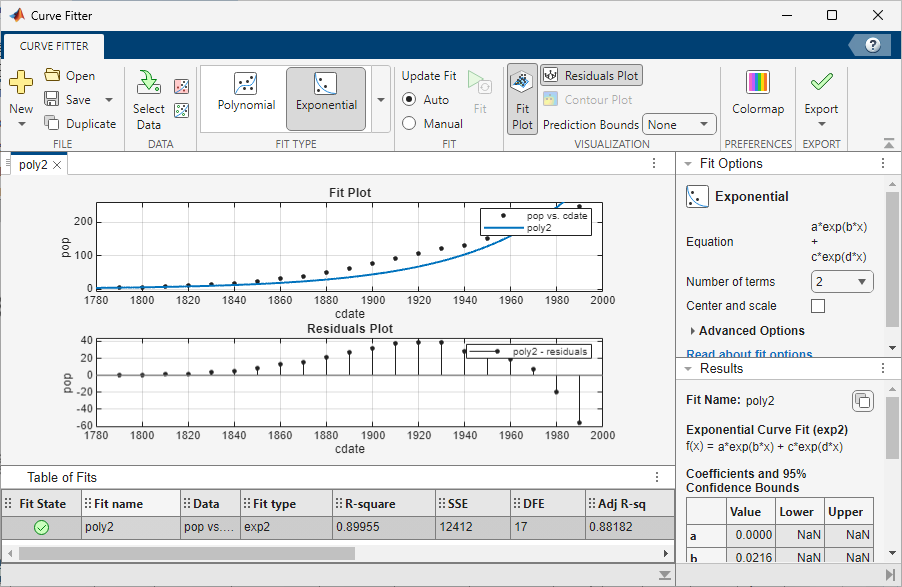# Curve Fitting

Fit curves and surfaces to data

## Description

The Curve Fitting app provides a flexible interface where you can interactively fit curves and surfaces to data and view plots.

You can:

• Create, plot, and compare multiple fits.

• Use linear or nonlinear regression, interpolation, smoothing, and custom equations.

• View goodness-of-fit statistics, display confidence intervals and residuals, remove outliers and assess fits with validation data.

• Automatically generate code to fit and plot curves and surfaces, or export fits to the workspace for further analysis.## Open the Curve Fitting App

• MATLAB® Toolstrip: On the Apps tab, under Math, Statistics and Optimization, click the app icon.

• MATLAB command prompt: Enter `cftool`.

## Programmatic Use

expand all

`cftool` opens Curve Fitting app or brings focus to the app if it is already open.

`cftool( x, y )` creates a curve fit to `x` input and `y` output. `x` and `y` must be numeric, have two or more elements, and have the same number of elements. `cftool` opens Curve Fitting app if necessary.

`cftool( x, y, z )` creates a surface fit to `x` and `y` inputs and `z` output. `x`, `y`, and `z` must be numeric, have two or more elements, and have compatible sizes. Sizes are compatible if `x`, `y`, and `z` all have the same number of elements or `x` and `y` are vectors, `z` is a 2D matrix, `length(x ) = n`, and `length(y) = m` where `[m,n] = size(z)`. `cftool` opens Curve Fitting app if necessary.

`cftool( x, y, [], w )` creates a curve fit with weights `w`. `w` must be numeric and have the same number of elements as `x` and `y`.

`cftool( x, y, z, w )` creates a surface fit with weights `w`. `w` must be numeric and have the same number of elements as `z`.

`cftool( filename )` loads the Curve Fitting session in `filename` into Curve Fitting app. The `filename` must have the extension `.sfit`.